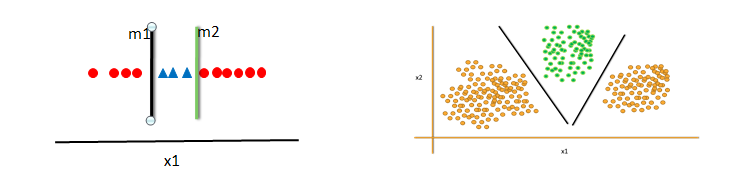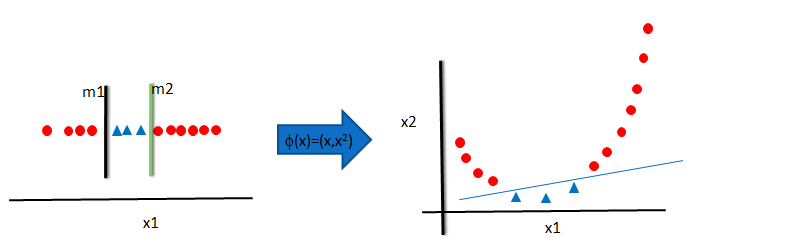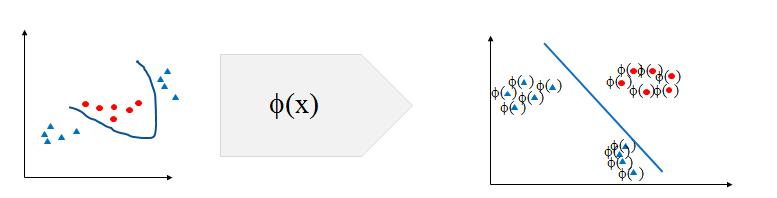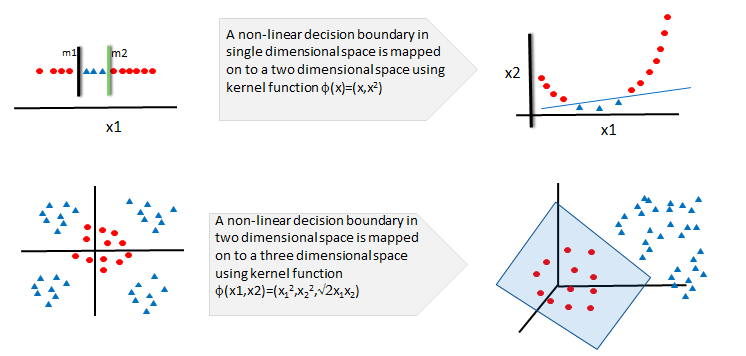• No products in the cart.

# 204.6.5 The Non-Linear Decision Boundary

##### What happens if data cannot be classified with a linear decision boundary.
Link to the previous post : https://statinfer.com/204-6-4-building-svm-model-in-python/

## The Non-Linear Decision Boundary

• In the above examples we can clearly see the decision boundary is linear.
• SVM works well when the data points are linearly separable.
• If the decision boundary is non-liner then SVM may struggle to classify.
• Observe the below examples, the classes are not linearly separable.
• SVM has no direct theory to set the non-liner decision boundary models.### Mapping to Higher Dimensional Space

• The original maximum-margin hyperplane algorithm proposed by Vapnik in 1963 constructed a linear classifier.
• To fit a non liner boundary classier, we can create new variables(dimensions) in the data and see whether the decision boundary is linear.
• In 1992, Bernhard E. Boser, Isabelle M. Guyon and Vladimir N. Vapnik suggested a way to create nonlinear classifiers by applying the kernel trick.
• In the below example, A single linear classifier is not sufficient.
• Lets create a new variable x2=(x1)2. In the higher dimensional space.
• We can clearly see a possibility of single linear decision boundary.
• This is called kernel trick.### Kernel Trick

• We used a function ϕ(x)=(x,(x2)) to transform the data x into a higher dimensional space.
• In the higher dimensional space, we could easily fit a liner decision boundary.
• This function ϕ(x) is known as kernel function and this process is known as kernel trick in SVM.• Kernel trick solves the non-linear decision boundary problem much like the hidden layers in neural networks.
• Kernel trick is simply increasing the number of dimensions. It is to make the non-linear decision boundary in lower dimensional space as a linear decision boundary, in higher dimensional space.
• In simple words, Kernel trick makes the non-linear decision boundary to linear (in higher dimensional space).### Kernel Function Examples

Name Function Type problem
Polynomial Kernelq is degree of polynomial Best for Image processing
Sigmoid Kernelk is offset value Very similar to neural network
Gaussian KernelNo prior knowledge on data
Linear KernelText Classification
Laplace Radial Basis Function (RBF)No prior knowledge on data
• There are many more kernel functions.

### Choosing the Kernel Function

• Probably the most tricky part of using SVM.
• The kernel function is important because it creates the kernel matrix, which summarizes all the data.
• There is no proven theory for choosing a kernel function for any given problem. Still there is lot of research going on.
• In practice, a low degree polynomial kernel or RBF kernel with a reasonable width is a good initial try.
• Choosing Kernel function is similar to choosing number of hidden layers in neural networks. Both of them have no proven theory to arrive at a standard value.
• As a first step, we can choose low degree polynomial or radial basis function or one of those from the list.

The next post is a practice session on kernel non-linear classifier.

Link to the next post : https://statinfer.com/204-6-6-practice-kernel-non-linear-classifier/

28th December 2017

### 0 responses on "204.6.5 The Non-Linear Decision Boundary"

Statinfer Software Solutions LLP

Software Technology Parks of India,
NH16, Krishna Nagar, Benz Circle,